# MF1_Lecture_01.ppt

Jun. 2, 2023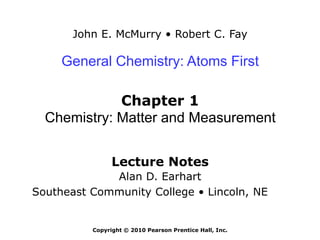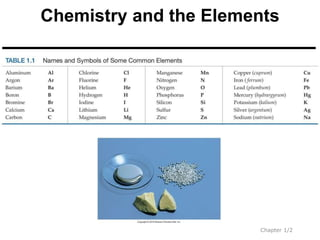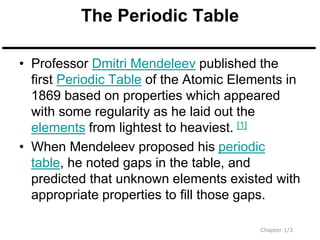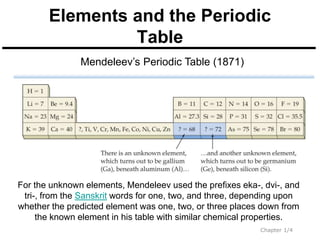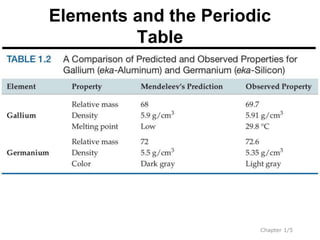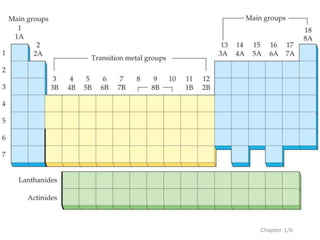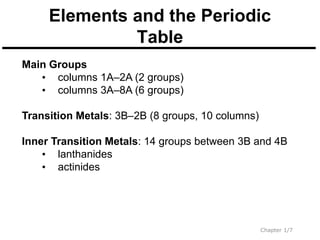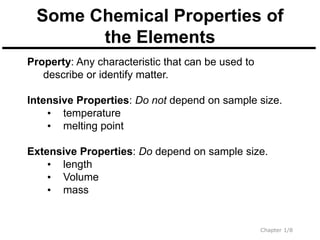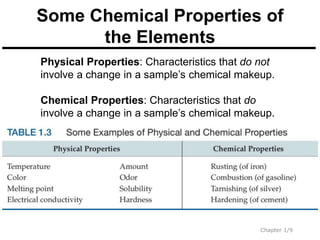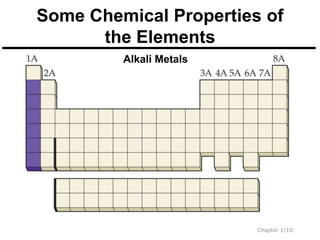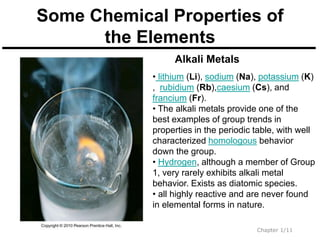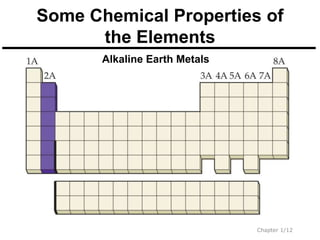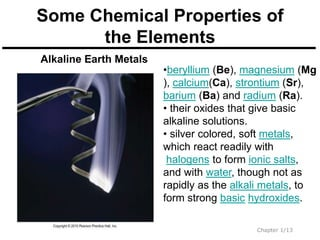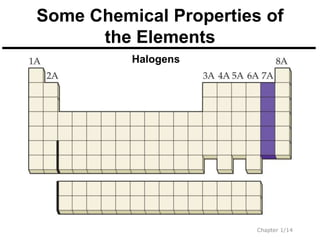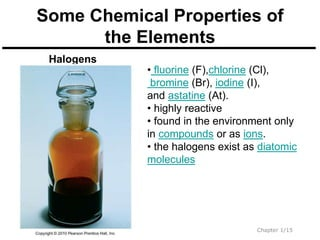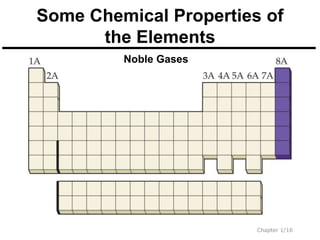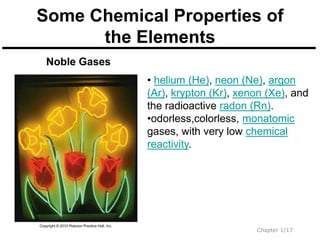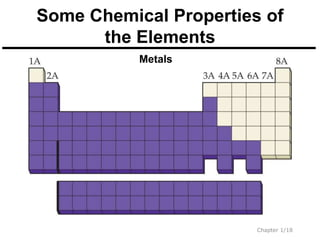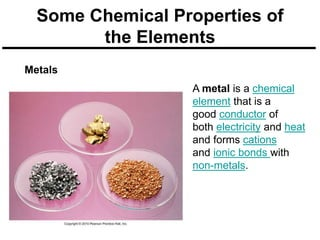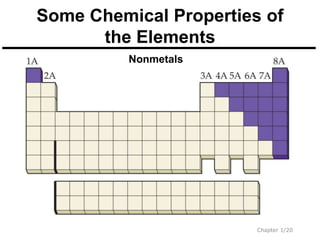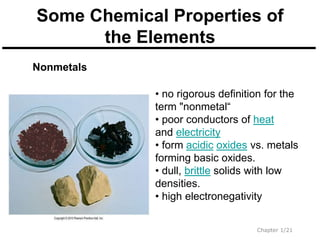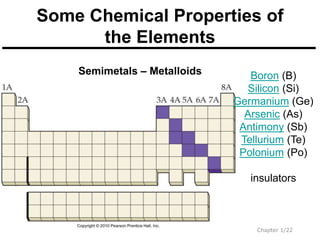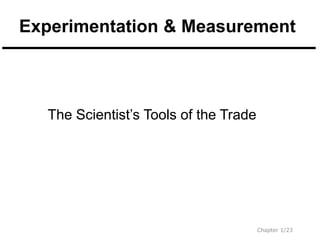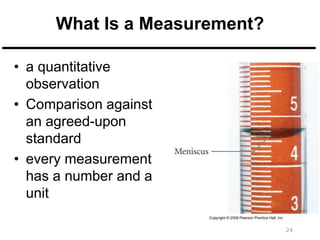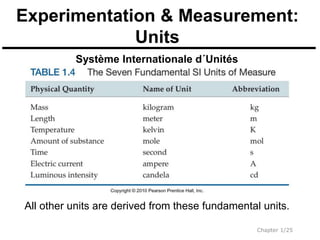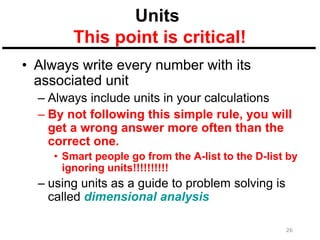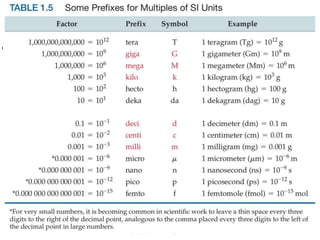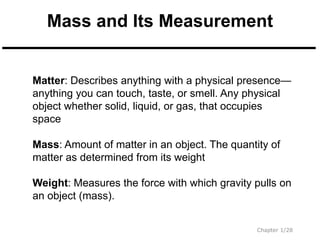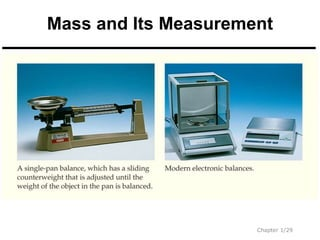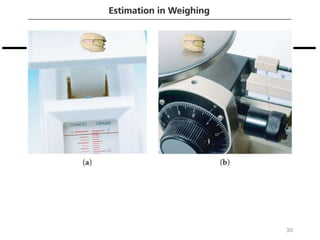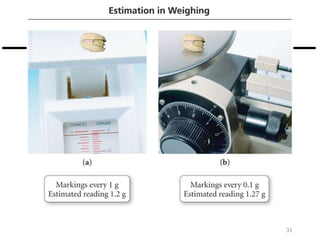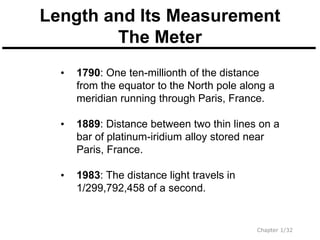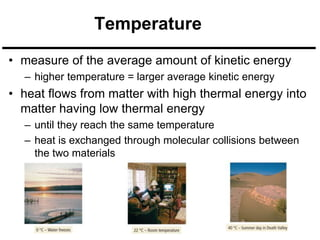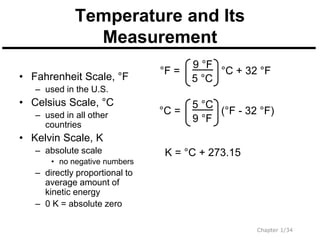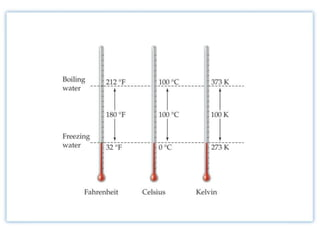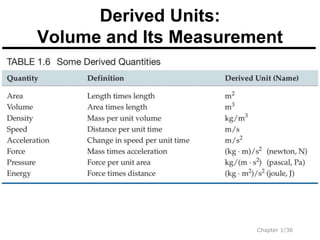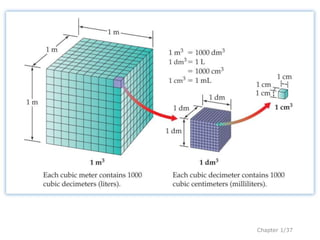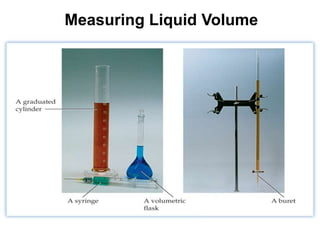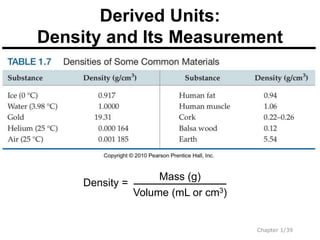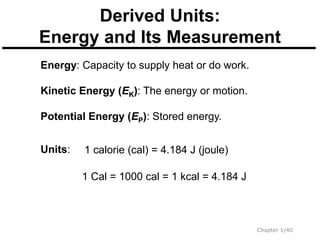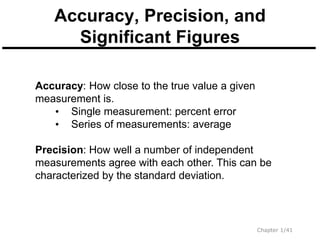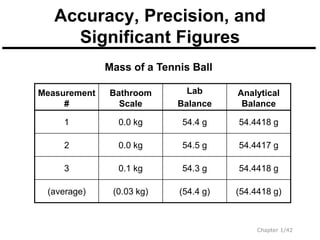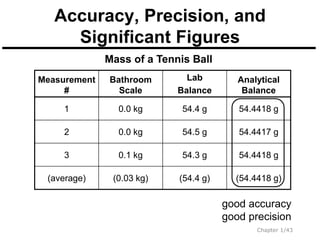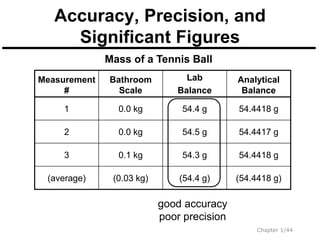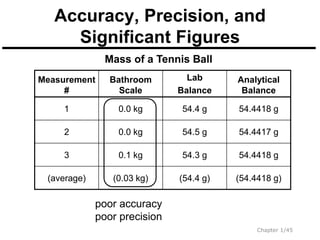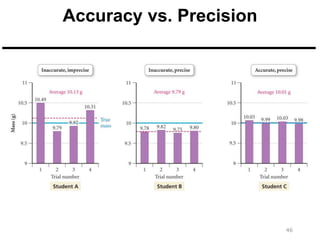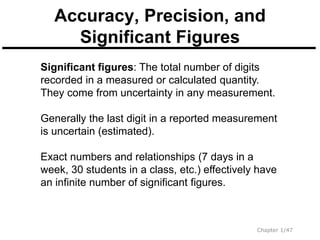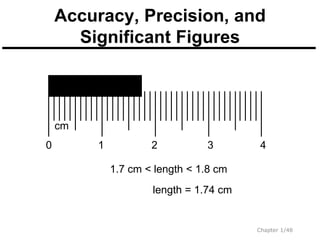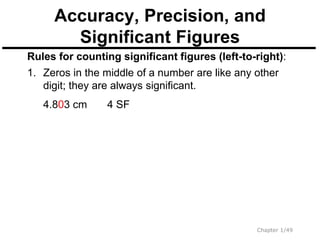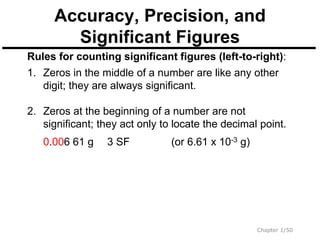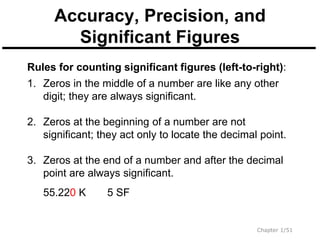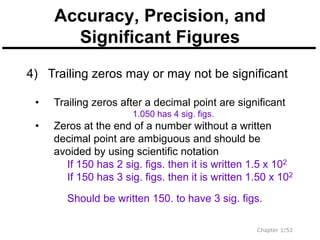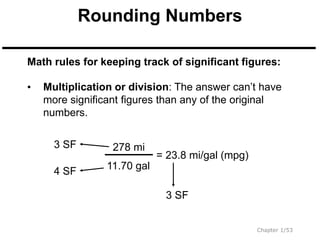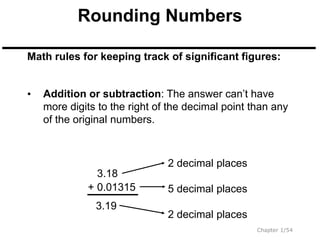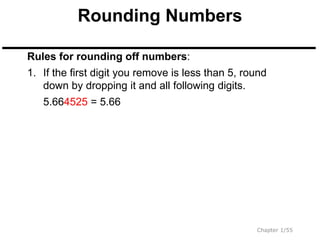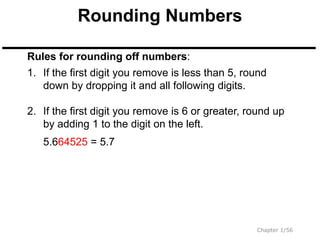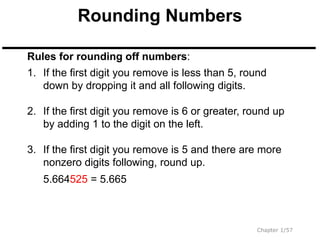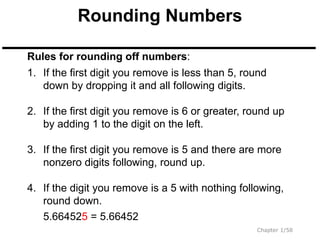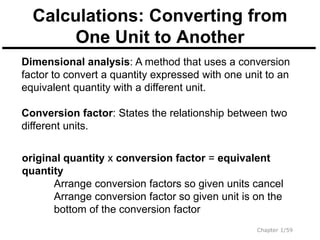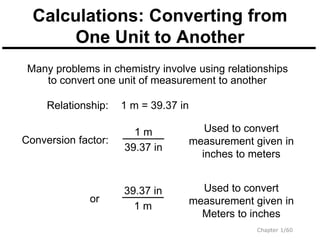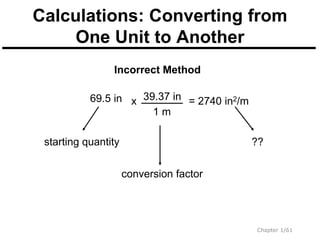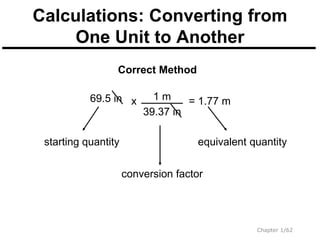1 of 62

### MF1_Lecture_01.ppt

• 1. John E. McMurry • Robert C. Fay Lecture Notes Alan D. Earhart Southeast Community College • Lincoln, NE General Chemistry: Atoms First Chapter 1 Chemistry: Matter and Measurement Copyright © 2010 Pearson Prentice Hall, Inc.
• 3. The Periodic Table • Professor Dmitri Mendeleev published the first Periodic Table of the Atomic Elements in 1869 based on properties which appeared with some regularity as he laid out the elements from lightest to heaviest.  • When Mendeleev proposed his periodic table, he noted gaps in the table, and predicted that unknown elements existed with appropriate properties to fill those gaps. Chapter 1/3
• 4. Chapter 1/4 Elements and the Periodic Table Mendeleev’s Periodic Table (1871) For the unknown elements, Mendeleev used the prefixes eka-, dvi-, and tri-, from the Sanskrit words for one, two, and three, depending upon whether the predicted element was one, two, or three places down from the known element in his table with similar chemical properties.
• 5. Chapter 1/5 Elements and the Periodic Table
• 7. Chapter 1/7 Elements and the Periodic Table Main Groups • columns 1A–2A (2 groups) • columns 3A–8A (6 groups) Transition Metals: 3B–2B (8 groups, 10 columns) Inner Transition Metals: 14 groups between 3B and 4B • lanthanides • actinides
• 8. Chapter 1/8 Some Chemical Properties of the Elements Property: Any characteristic that can be used to describe or identify matter. Intensive Properties: Do not depend on sample size. • temperature • melting point Extensive Properties: Do depend on sample size. • length • Volume • mass
• 9. Chapter 1/9 Some Chemical Properties of the Elements Physical Properties: Characteristics that do not involve a change in a sample’s chemical makeup. Chemical Properties: Characteristics that do involve a change in a sample’s chemical makeup.
• 10. Chapter 1/10 Some Chemical Properties of the Elements Alkali Metals
• 11. Chapter 1/11 Some Chemical Properties of the Elements Alkali Metals • lithium (Li), sodium (Na), potassium (K) , rubidium (Rb),caesium (Cs), and francium (Fr). • The alkali metals provide one of the best examples of group trends in properties in the periodic table, with well characterized homologous behavior down the group. • Hydrogen, although a member of Group 1, very rarely exhibits alkali metal behavior. Exists as diatomic species. • all highly reactive and are never found in elemental forms in nature.
• 12. Chapter 1/12 Some Chemical Properties of the Elements Alkaline Earth Metals
• 13. Chapter 1/13 Some Chemical Properties of the Elements Alkaline Earth Metals •beryllium (Be), magnesium (Mg ), calcium(Ca), strontium (Sr), barium (Ba) and radium (Ra). • their oxides that give basic alkaline solutions. • silver colored, soft metals, which react readily with halogens to form ionic salts, and with water, though not as rapidly as the alkali metals, to form strong basic hydroxides.
• 14. Chapter 1/14 Some Chemical Properties of the Elements Halogens
• 15. Chapter 1/15 Some Chemical Properties of the Elements Halogens • fluorine (F),chlorine (Cl), bromine (Br), iodine (I), and astatine (At). • highly reactive • found in the environment only in compounds or as ions. • the halogens exist as diatomic molecules
• 16. Chapter 1/16 Some Chemical Properties of the Elements Noble Gases
• 17. Chapter 1/17 Some Chemical Properties of the Elements Noble Gases • helium (He), neon (Ne), argon (Ar), krypton (Kr), xenon (Xe), and the radioactive radon (Rn). •odorless,colorless, monatomic gases, with very low chemical reactivity.
• 18. Chapter 1/18 Some Chemical Properties of the Elements Metals
• 19. Some Chemical Properties of the Elements Metals A metal is a chemical element that is a good conductor of both electricity and heat and forms cations and ionic bonds with non-metals.
• 20. Chapter 1/20 Some Chemical Properties of the Elements Nonmetals
• 21. Chapter 1/21 Some Chemical Properties of the Elements Nonmetals • no rigorous definition for the term "nonmetal“ • poor conductors of heat and electricity • form acidic oxides vs. metals forming basic oxides. • dull, brittle solids with low densities. • high electronegativity
• 22. Chapter 1/22 Some Chemical Properties of the Elements Semimetals – Metalloids Boron (B) Silicon (Si) Germanium (Ge) Arsenic (As) Antimony (Sb) Tellurium (Te) Polonium (Po) insulators
• 23. Experimentation & Measurement Chapter 1/23 The Scientist’s Tools of the Trade
• 24. 24 What Is a Measurement? • a quantitative observation • Comparison against an agreed-upon standard • every measurement has a number and a unit
• 25. Chapter 1/25 Experimentation & Measurement: Units Système Internationale d´Unités All other units are derived from these fundamental units.
• 26. 26 Units This point is critical! • Always write every number with its associated unit – Always include units in your calculations – By not following this simple rule, you will get a wrong answer more often than the correct one. • Smart people go from the A-list to the D-list by ignoring units!!!!!!!!!! – using units as a guide to problem solving is called dimensional analysis
• 28. Chapter 1/28 Mass and Its Measurement Matter: Describes anything with a physical presence— anything you can touch, taste, or smell. Any physical object whether solid, liquid, or gas, that occupies space Mass: Amount of matter in an object. The quantity of matter as determined from its weight Weight: Measures the force with which gravity pulls on an object (mass).
• 29. Chapter 1/29 Mass and Its Measurement
• 30. 30
• 31. 31
• 32. Chapter 1/32 Length and Its Measurement The Meter • 1790: One ten-millionth of the distance from the equator to the North pole along a meridian running through Paris, France. • 1889: Distance between two thin lines on a bar of platinum-iridium alloy stored near Paris, France. • 1983: The distance light travels in 1/299,792,458 of a second.
• 33. Temperature • measure of the average amount of kinetic energy – higher temperature = larger average kinetic energy • heat flows from matter with high thermal energy into matter having low thermal energy – until they reach the same temperature – heat is exchanged through molecular collisions between the two materials
• 34. Chapter 1/34 Temperature and Its Measurement (°F - 32 °F) 5 °C 9 °F °C = °C + 32 °F 9 °F 5 °C °F = K = °C + 273.15 • Fahrenheit Scale, °F – used in the U.S. • Celsius Scale, °C – used in all other countries • Kelvin Scale, K – absolute scale • no negative numbers – directly proportional to average amount of kinetic energy – 0 K = absolute zero
• 36. Chapter 1/36 Derived Units: Volume and Its Measurement
• 39. Chapter 1/39 Derived Units: Density and Its Measurement Density = Volume (mL or cm3) Mass (g)
• 40. Chapter 1/40 Derived Units: Energy and Its Measurement Energy: Capacity to supply heat or do work. Kinetic Energy (EK): The energy or motion. Potential Energy (EP): Stored energy. Units: 1 Cal = 1000 cal = 1 kcal = 4.184 J 1 calorie (cal) = 4.184 J (joule)
• 41. Chapter 1/41 Accuracy, Precision, and Significant Figures Accuracy: How close to the true value a given measurement is. • Single measurement: percent error • Series of measurements: average Precision: How well a number of independent measurements agree with each other. This can be characterized by the standard deviation.
• 42. Chapter 1/42 Accuracy, Precision, and Significant Figures Mass of a Tennis Ball Measurement # Bathroom Scale Lab Balance Analytical Balance 1 0.0 kg 54.4 g 54.4418 g 2 0.0 kg 54.5 g 54.4417 g 3 0.1 kg 54.3 g 54.4418 g (average) (0.03 kg) (54.4 g) (54.4418 g)
• 43. Chapter 1/43 Accuracy, Precision, and Significant Figures Measurement # Bathroom Scale Lab Balance Analytical Balance 1 0.0 kg 54.4 g 54.4418 g 2 0.0 kg 54.5 g 54.4417 g 3 0.1 kg 54.3 g 54.4418 g (average) (0.03 kg) (54.4 g) (54.4418 g) good accuracy good precision Mass of a Tennis Ball
• 44. Chapter 1/44 Accuracy, Precision, and Significant Figures Measurement # Bathroom Scale Lab Balance Analytical Balance 1 0.0 kg 54.4 g 54.4418 g 2 0.0 kg 54.5 g 54.4417 g 3 0.1 kg 54.3 g 54.4418 g (average) (0.03 kg) (54.4 g) (54.4418 g) good accuracy poor precision Mass of a Tennis Ball
• 45. Chapter 1/45 Accuracy, Precision, and Significant Figures Measurement # Bathroom Scale Lab Balance Analytical Balance 1 0.0 kg 54.4 g 54.4418 g 2 0.0 kg 54.5 g 54.4417 g 3 0.1 kg 54.3 g 54.4418 g (average) (0.03 kg) (54.4 g) (54.4418 g) poor accuracy poor precision Mass of a Tennis Ball
• 47. Chapter 1/47 Accuracy, Precision, and Significant Figures Significant figures: The total number of digits recorded in a measured or calculated quantity. They come from uncertainty in any measurement. Generally the last digit in a reported measurement is uncertain (estimated). Exact numbers and relationships (7 days in a week, 30 students in a class, etc.) effectively have an infinite number of significant figures.
• 48. Chapter 1/48 Accuracy, Precision, and Significant Figures length = 1.74 cm 0 1 2 4 3 cm 1.7 cm < length < 1.8 cm
• 49. Chapter 1/49 Accuracy, Precision, and Significant Figures 4.803 cm 4 SF Rules for counting significant figures (left-to-right): 1. Zeros in the middle of a number are like any other digit; they are always significant.
• 50. Chapter 1/50 Accuracy, Precision, and Significant Figures 0.006 61 g 3 SF (or 6.61 x 10-3 g) Rules for counting significant figures (left-to-right): 1. Zeros in the middle of a number are like any other digit; they are always significant. 2. Zeros at the beginning of a number are not significant; they act only to locate the decimal point.
• 51. Chapter 1/51 Accuracy, Precision, and Significant Figures 55.220 K 5 SF Rules for counting significant figures (left-to-right): 1. Zeros in the middle of a number are like any other digit; they are always significant. 2. Zeros at the beginning of a number are not significant; they act only to locate the decimal point. 3. Zeros at the end of a number and after the decimal point are always significant.
• 52. Chapter 1/52 Accuracy, Precision, and Significant Figures 4) Trailing zeros may or may not be significant • Trailing zeros after a decimal point are significant 1.050 has 4 sig. figs. • Zeros at the end of a number without a written decimal point are ambiguous and should be avoided by using scientific notation If 150 has 2 sig. figs. then it is written 1.5 x 102 If 150 has 3 sig. figs. then it is written 1.50 x 102 Should be written 150. to have 3 sig. figs.
• 53. Chapter 1/53 Rounding Numbers Math rules for keeping track of significant figures: • Multiplication or division: The answer can’t have more significant figures than any of the original numbers. 11.70 gal 278 mi = 23.8 mi/gal (mpg) 4 SF 3 SF 3 SF
• 54. Chapter 1/54 Rounding Numbers Math rules for keeping track of significant figures: • Addition or subtraction: The answer can’t have more digits to the right of the decimal point than any of the original numbers. 3.19 + 0.01315 3.18 2 decimal places 5 decimal places 2 decimal places
• 55. Chapter 1/55 Rounding Numbers Rules for rounding off numbers: 1. If the first digit you remove is less than 5, round down by dropping it and all following digits. 5.664525 = 5.66
• 56. Chapter 1/56 Rounding Numbers Rules for rounding off numbers: 1. If the first digit you remove is less than 5, round down by dropping it and all following digits. 2. If the first digit you remove is 6 or greater, round up by adding 1 to the digit on the left. 5.664525 = 5.7
• 57. Chapter 1/57 Rounding Numbers Rules for rounding off numbers: 1. If the first digit you remove is less than 5, round down by dropping it and all following digits. 2. If the first digit you remove is 6 or greater, round up by adding 1 to the digit on the left. 3. If the first digit you remove is 5 and there are more nonzero digits following, round up. 5.664525 = 5.665
• 58. Chapter 1/58 Rounding Numbers Rules for rounding off numbers: 1. If the first digit you remove is less than 5, round down by dropping it and all following digits. 2. If the first digit you remove is 6 or greater, round up by adding 1 to the digit on the left. 3. If the first digit you remove is 5 and there are more nonzero digits following, round up. 4. If the digit you remove is a 5 with nothing following, round down. 5.664525 = 5.66452
• 59. Chapter 1/59 Calculations: Converting from One Unit to Another Dimensional analysis: A method that uses a conversion factor to convert a quantity expressed with one unit to an equivalent quantity with a different unit. Conversion factor: States the relationship between two different units. original quantity x conversion factor = equivalent quantity Arrange conversion factors so given units cancel Arrange conversion factor so given unit is on the bottom of the conversion factor
• 60. Chapter 1/60 Calculations: Converting from One Unit to Another 1 m = 39.37 in Conversion factor: Relationship: 1 m 39.37 in or 39.37 in 1 m Used to convert measurement given in inches to meters Used to convert measurement given in Meters to inches Many problems in chemistry involve using relationships to convert one unit of measurement to another
• 61. Chapter 1/61 Calculations: Converting from One Unit to Another 1 m 39.37 in 69.5 in = 2740 in2/m x ?? starting quantity conversion factor Incorrect Method
• 62. Chapter 1/62 Calculations: Converting from One Unit to Another 39.37 in 1 m 69.5 in = 1.77 m x equivalent quantity starting quantity conversion factor Correct Method